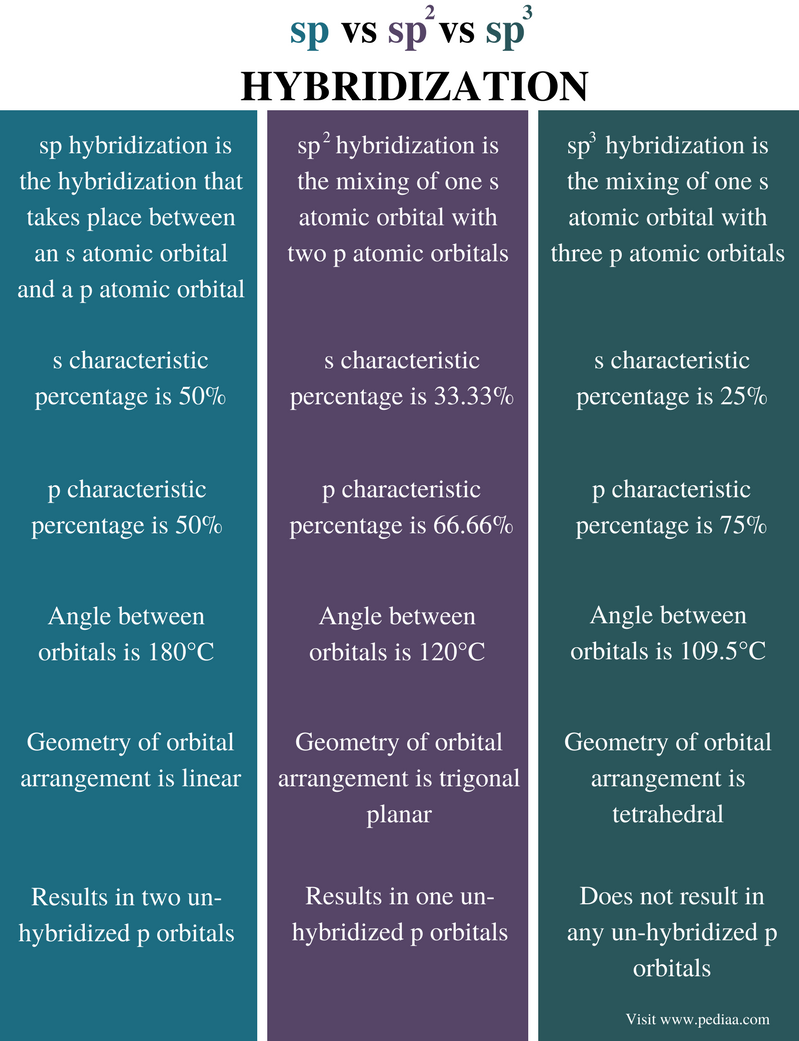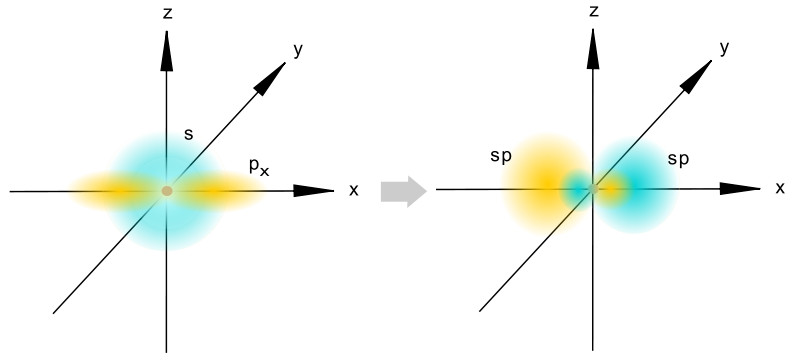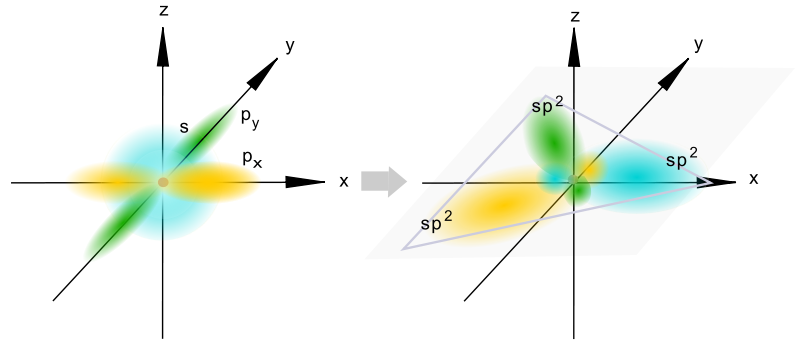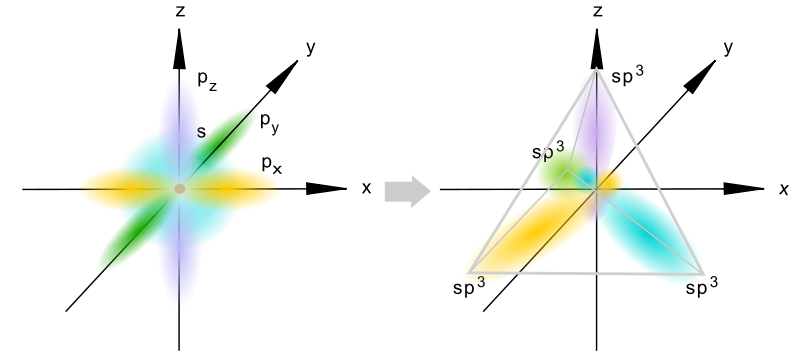# Difference Between sp sp2 and sp3 Hybridization

## Main Difference – sp vs sp2 vs sp3 Hybridization

Orbitals are hypothetical structures that can be filled with electrons. According to different discoveries, scientists have proposed different shapes for these orbitals. There are three main types of orbitals: atomic orbitals, molecular orbitals, and hybrid orbitals. Atomic orbitals of an atom undergo hybridization to make suitable orbitals for chemical bonding. In chemistry, hybridization is the mixing of different atomic orbitals to form hybrid orbitals. There are different forms of hybridizations which make different forms of hybrid orbitals such as sp, sp2 and sp3 hybrid orbitals. These orbitals are formed by the hybridization of s and p atomic orbitals in different ratios. The main difference between sp sp2 and sp3 hybridization is that sp hybridization forms hybrid orbitals having 50% s orbital characteristics and sp2 hybridization forms hybrid orbitals having 33% s orbital characteristics whereas sp3 hybridization forms hybrid orbitals having 25% s orbital characteristics.

### Key Areas Covered

1. What is sp Hybridization
– Definition, Calculation of S and P Characteristics, Other Features
2. What is sp2 Hybridization
– Definition, Calculation of S and P Characteristics, Other Features
3. What is sp3 Hybridization
– Definition, Calculation of S and P Characteristics, Other Features
4. What is the Difference Between sp sp2 and sp3 Hybridization
– Comparison of Key Differences

Key Terms: Atomic Orbital, Hybrid Orbitals, Hybridization, Orbitals, Sp Hybridization, Sp2 Hybridization, Sp3 Hybridization## What is sp Hybridization

sp hybridization is the hybridization that takes place between an s atomic orbital and a p atomic orbital. An electron shell contains three p orbitals. Therefore, after the hybridization of an s orbital with one of these p orbitals, there are two un-hybridized p orbitals present in that atom. Here, we consider that all s and p orbitals as just atomic orbitals (s+p). The ratio between s and p orbitals is 1:1. Therefore the fraction of s orbital is 1/2, and the fraction of p orbitals is 1/2.

S (or p) characteristic percentage = total atomic orbitals  x  (1/2)  x  100%
= 50%Figure 1: sp Hybridization

The resulting hybrid orbitals have 50% of s characteristics and 50% of p characteristics. Since only two hybrid orbitals formed, the spatial arrangement of sp orbitals is linear. The two hybrid orbitals are directed to opposite directions. Hence, the angle between these orbitals is 180oC.

## What is sp2 Hybridization

sp2 hybridization is the mixing of one s atomic orbital with two p atomic orbitals. The newly formed hybrid orbitals are known as sp2 hybrid orbitals. The resulting hybrid orbitals have about 33.33% of s characters and about 66.66% of p characters. This is because a total of three atomic orbitals are involved in the hybridization, and the percentages of s and p characteristics vary as follows.

Here, we consider that all s and p orbitals as just atomic orbitals (s+p+p). The ratio between s and p orbitals is 1:2. Therefore the fraction of s orbital is 1/3 and the fraction of p orbitals is 2/3.

S characteristic percentage = total atomic orbitals  x  (1/ 3)  x  100%
= 33.33 %
P characteristic percentage = total atomic orbitals  x  (2/ 3)  x  100%
= 66.66 %Figure 2: sp2 Hybridization

The spatial arrangement of sp2 hybrid orbitals is trigonal planar. Therefore, the angle between these orbitals is 120oC. The atoms that undergo this hybridization have 1 un-hybridized p orbital because only two of three p orbitals are involved in this hybridization.

## What is sp3 Hybridization

sp3 Hybridization is the mixing of one s atomic orbital with three p atomic orbitals. Here, the atoms do not have un-hybridized p orbitals because all three p orbitals are involved in hybridization. The resulting orbitals are known as sp3 hybrid orbitals. As same as for sporbitals, we can calculate the s and p characteristics of these orbitals.

In sp3 hybridization, we consider that all s and p orbitals as just atomic orbitals (s+p+p+p). The ratio between s and p orbitals is 1:3. Therefore the fraction of s orbital is ¼, and the fraction of p orbitals is ¾.

S characteristic percentage = total atomic orbitals  x  (1/4) x  100%
= 25 %
p characteristic percentage = total atomic orbitals  x  (3/4)  x  100%
= 75 %Figure 3: sp3 Hybridization

These orbitals are formed when one s orbital, and 3 p orbitals are hybridized. The resulting hybrid orbitals have about 25% of s characters and about 75% of p characters. The spatial arrangement of these orbitals is tetrahedral. Therefore, the angle between these orbitals is 109.5oC.

## Difference Between sp sp2 and sp3 Hybridization

### Definition

sp Hybridization: sp hybridization is the hybridization that takes place between an s atomic orbital and a p atomic orbital.

sp2 Hybridization: sp2 hybridization is the mixing of one s atomic orbital with two p atomic orbitals.

sp3 Hybridization: sp3 hybridization is the mixing of one s atomic orbital with three p atomic orbitals.

### S Characteristics

sp Hybridization: The s characteristic percentage of sp hybrid orbitals is 50%.

sp2 Hybridization: The s characteristic percentage of sp2 hybrid orbitals is 33.33%.

sp3 Hybridization: The s characteristic percentage of sp3 hybrid orbitals is 25%.

### P Characteristic Percentage of Hybrid Orbitals

sp Hybridization: The p characteristic percentage of sp hybrid orbitals is 50%.

sp2 Hybridization: The p characteristic percentage of sp2 hybrid orbitals is 66.66%.

sp3 Hybridization: The p characteristic percentage of sphybrid orbitals is 75%.

### Angle between Orbitals

sp Hybridization: The angle between sp orbitals is 180°C.

sp2 Hybridization: The angle between sporbitals is 120°C.

sp3 Hybridization: The angle between sporbitals is 109.5°C.

### Geometry

sp Hybridization: The geometry of orbital arrangement in sp hybridization is linear.

sp2 Hybridization: The geometry of orbital arrangement in sphybridization is trigonal planar.

sp3 Hybridization: The geometry of orbital arrangement in sphybridization is tetrahedral.

### Number of Un-hybridized Orbitals

sp Hybridization: Sp hybridization results in two un-hybridized p orbitals.

sp2 Hybridization: Sp2 hybridization results in one un-hybridized p orbitals.

sp3 Hybridization: Sp3 hybridization does not result in any un-hybridized p orbitals.

### Conclusion

Hybridization in chemistry stands for the mixing of different atomic orbitals to form new hybrid orbitals with different characteristics. Sp, sp2 and sp3 hybridizations are such examples. The main difference between sp, sp2 and sp3 hybridization is that sp hybridization forms hybrid orbitals having 50% s orbital characteristics and sp2 hybridization forms hybrid orbitals having 33% s orbital characteristics whereas sp3 hybridization forms hybrid orbitals having 25% s orbital characteristics.

##### Reference:

1.  “Hybrid Orbitals.” Chemistry LibreTexts, Libretexts, 21 July 2016, Available here.
2. “Orbital hybridisation.” Wikipedia, Wikimedia Foundation, 15 Jan. 2018, Available here.

##### Image Courtesy:

1. “Hybrydyzacja sp” By Joanna Kośmider – Own work (Public Domain) via Commons Wikimedia
2. “Hybrydyzacja sp2” By Joanna Kośmider – Own work (Public Domain) via Commons Wikimedia
3. “Hybrydyzacja sp3” By Joanna Kośmider – Own work (Public Domain) via Commons Wikimedia